# RD Sharma Solutions Class 11 Geometric Progressions Exercise 20.3

This Exercise of RD Sharma Class 11 Chapter 20 discusses problems based on the sum of the terms of a G.P, with examples for better understanding. For students who aim to excel in the Class 11 Mathematics examinations, a proper methodology of understanding the concepts and solving problems is a must. The RD Sharma Class 11 Maths Solutions is on par with these requirements, for a student to secure good marks in the board exams. The solutions here have been developed by our subject expert team at BYJU’S, in simple language along with appropriate examples and explanations, to give clarity on the concepts. To know more about this exercise, students can download the pdf of RD Sharma Solutions from the below-mentioned links.

## Download the pdf of RD Sharma Solutions for Class 11 Maths Exercise 20.3 Chapter 20 – Geometric Progression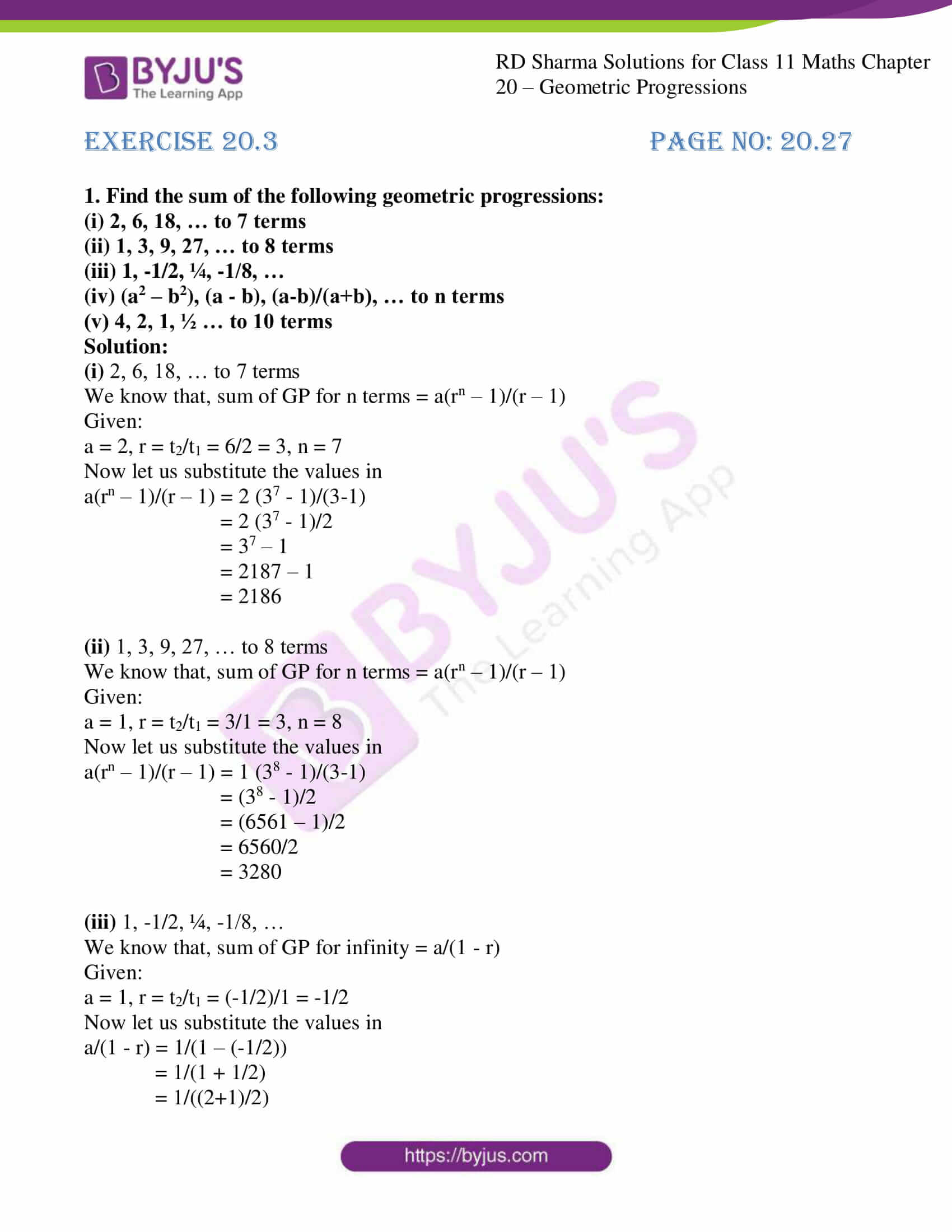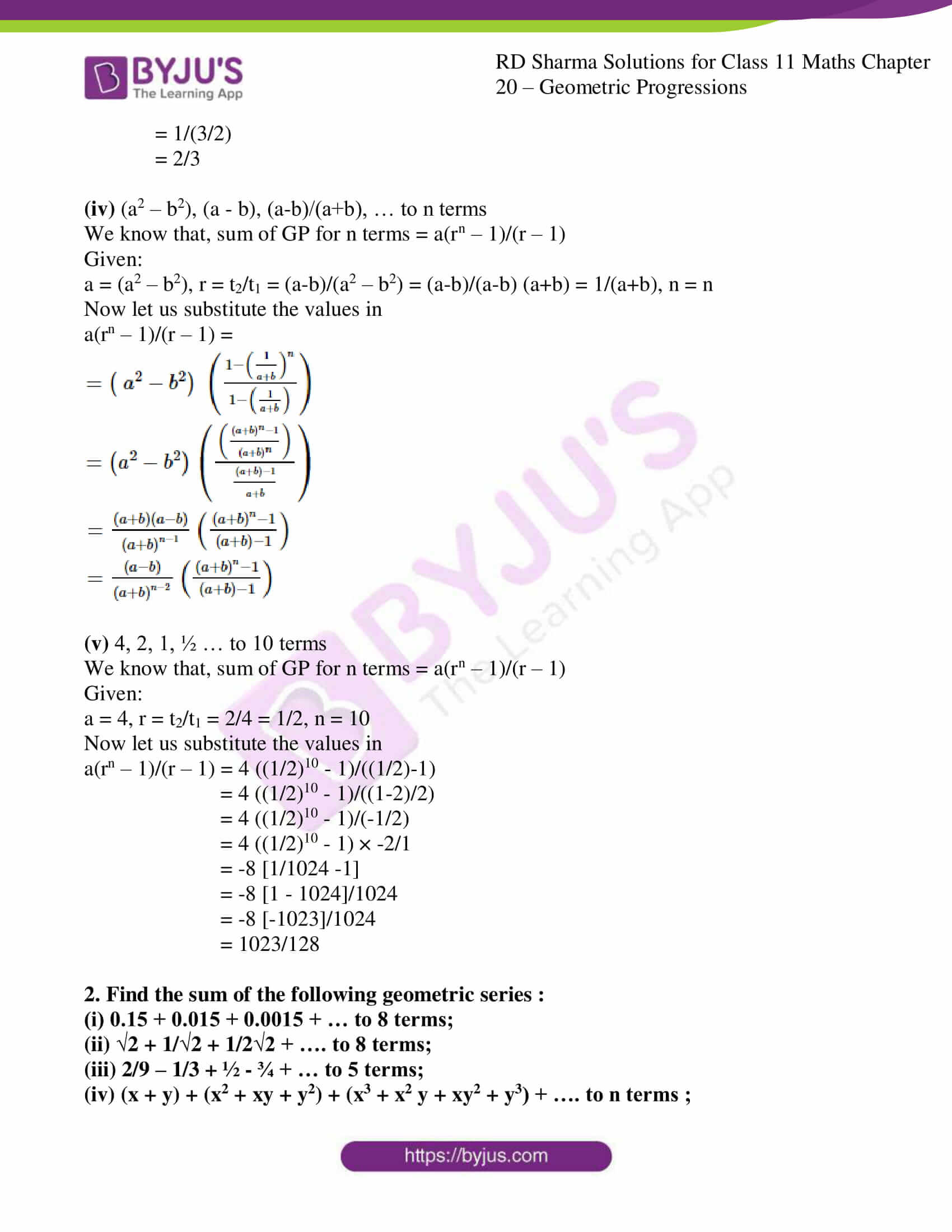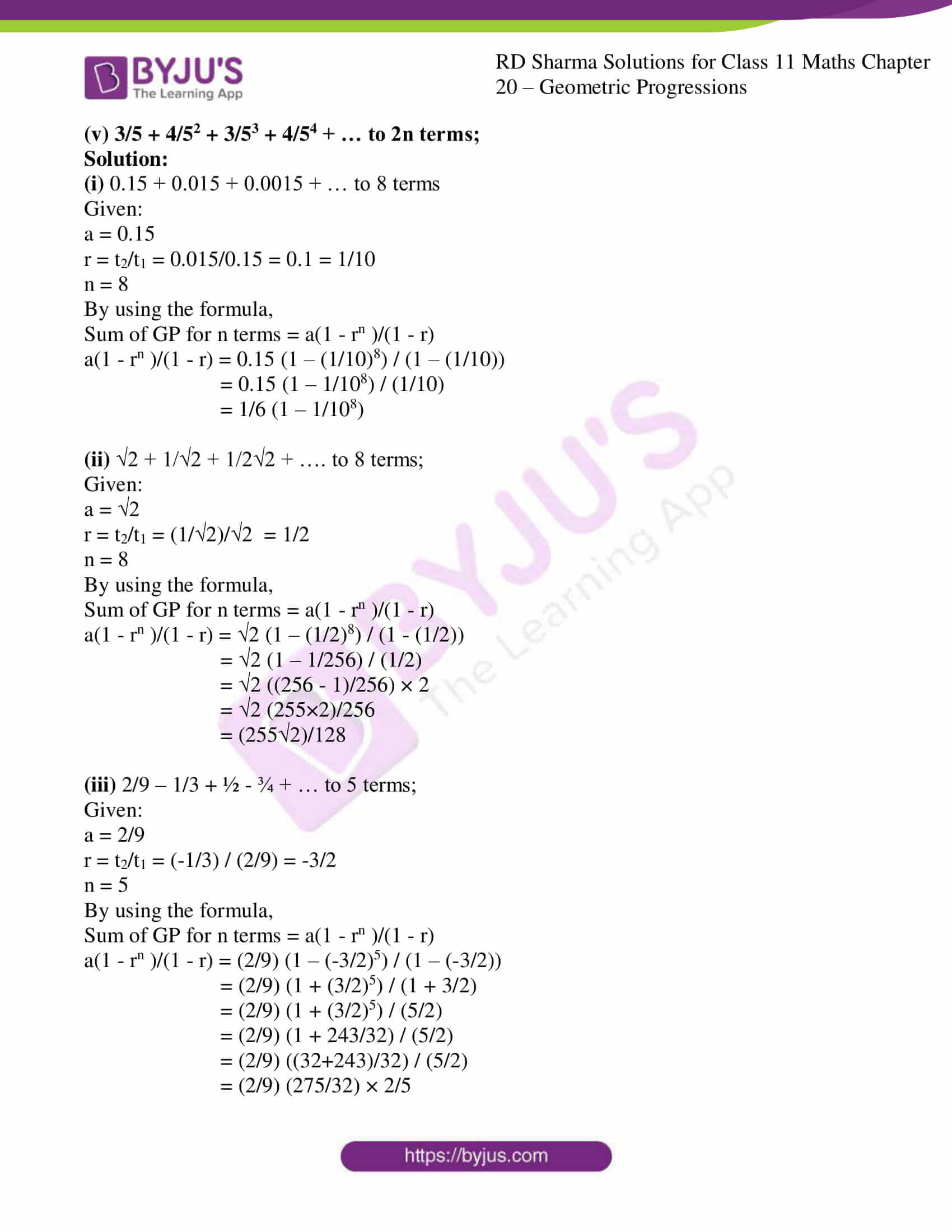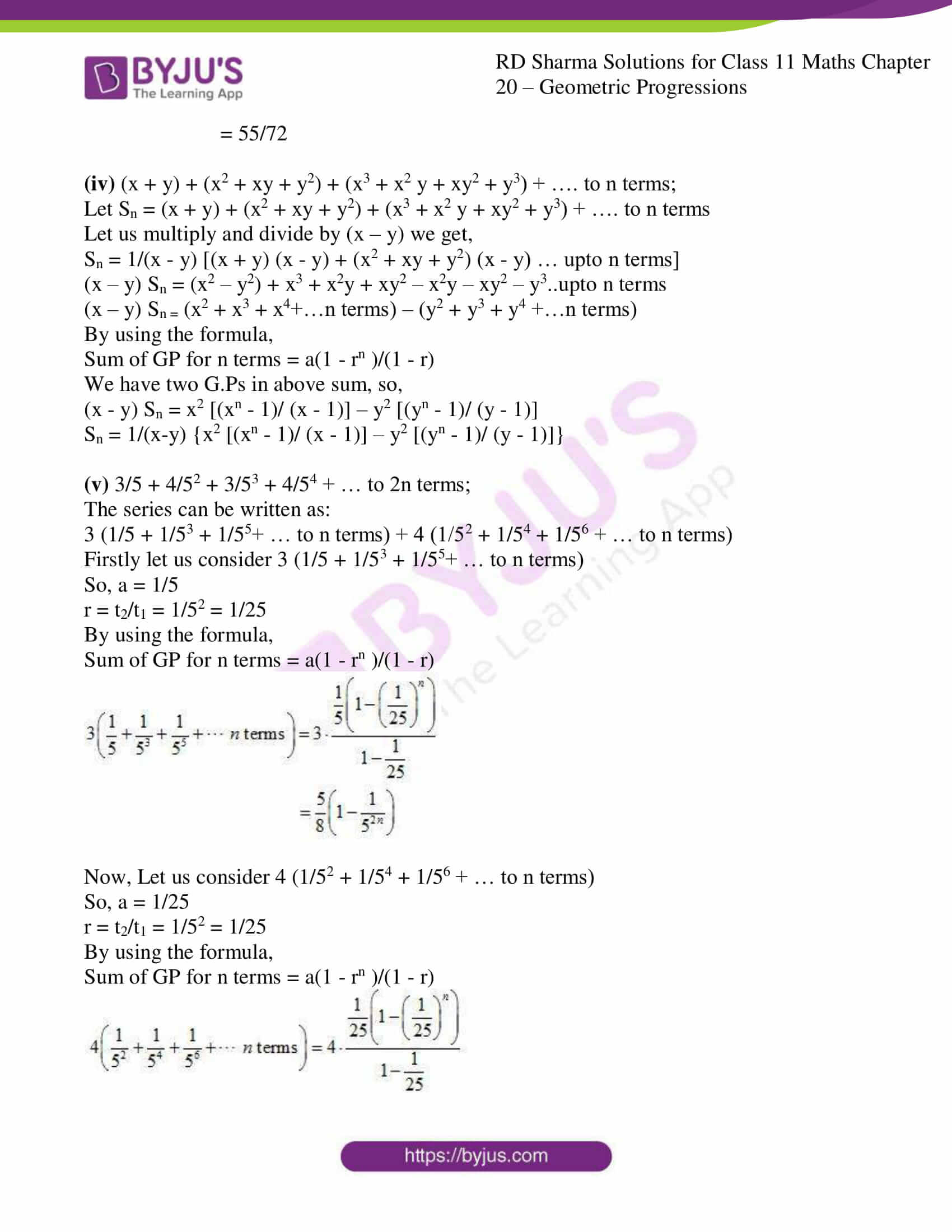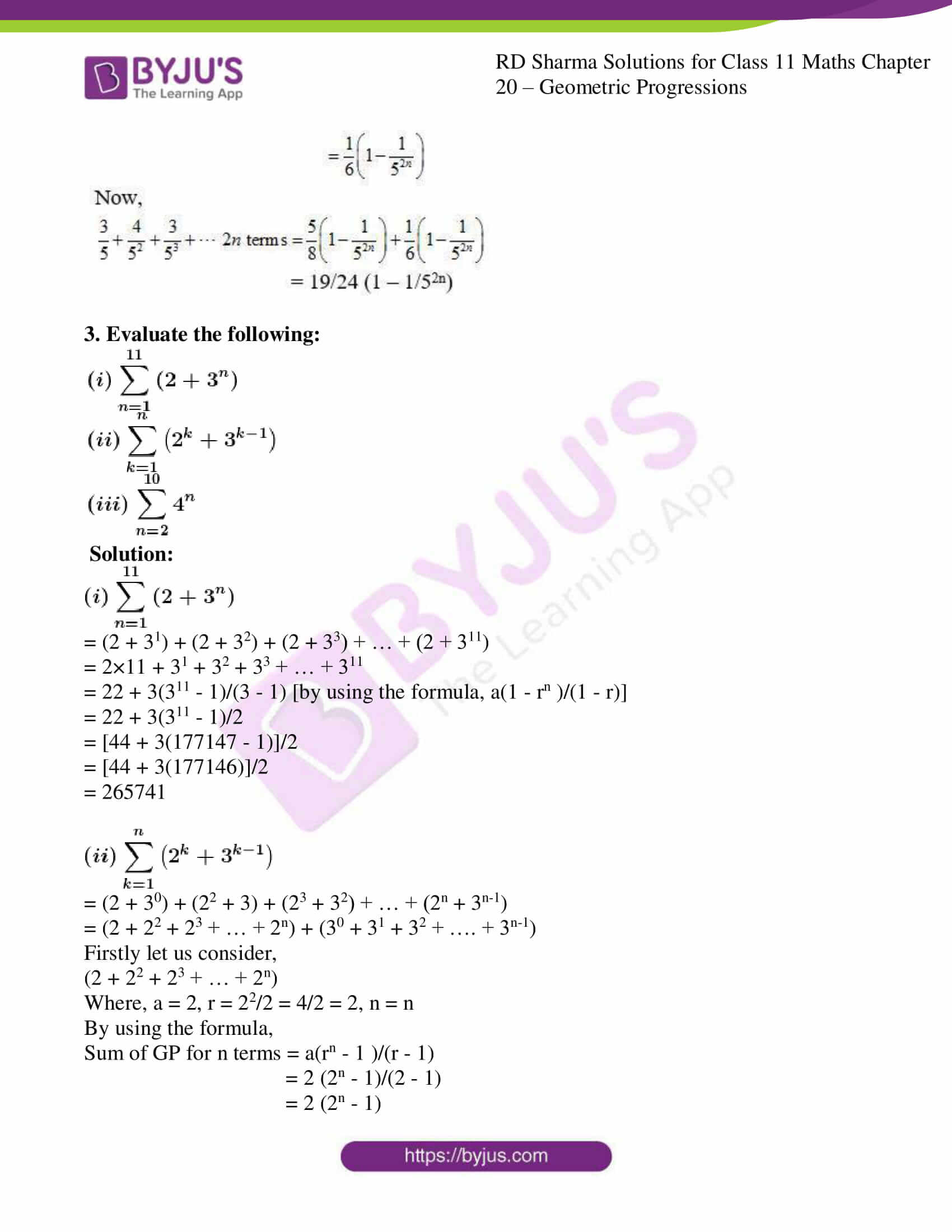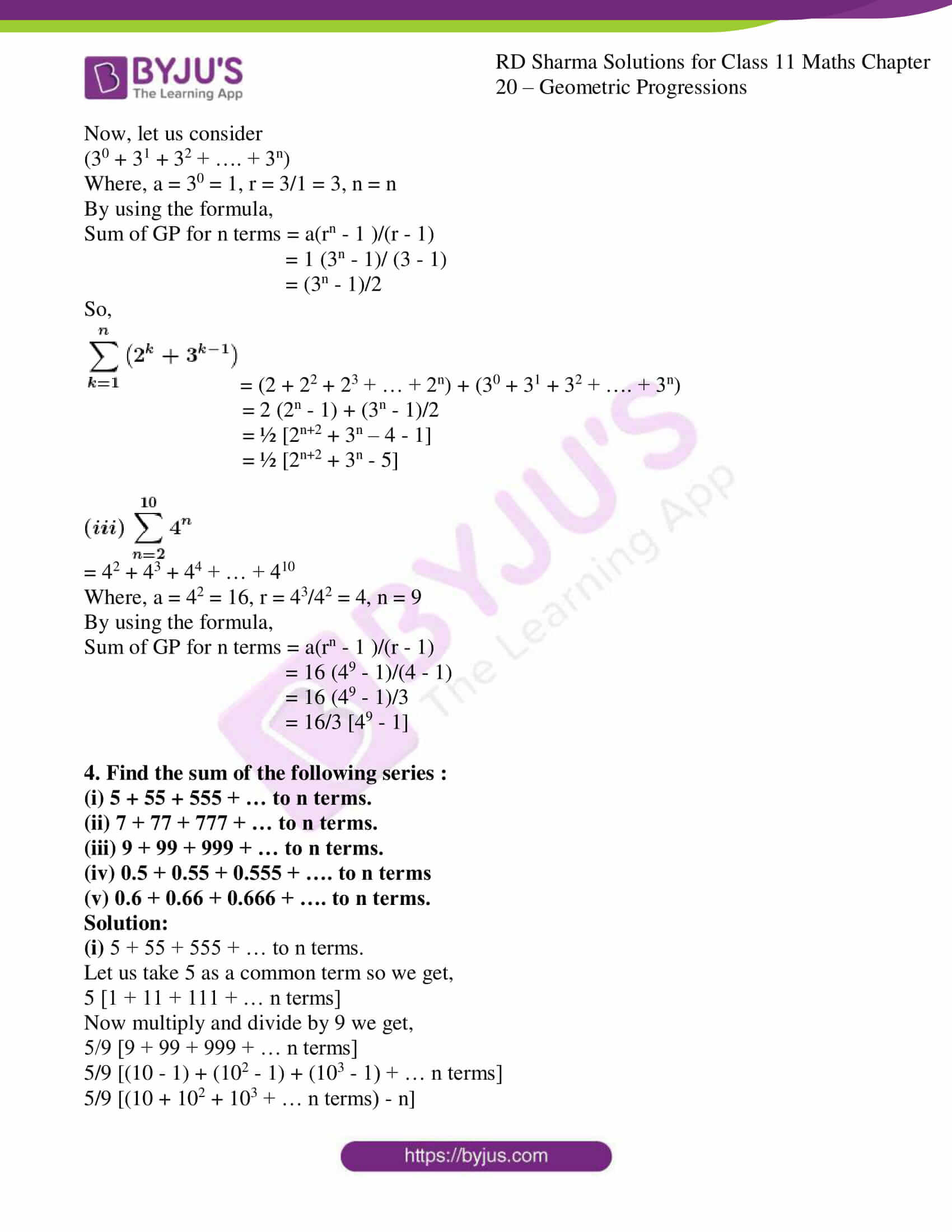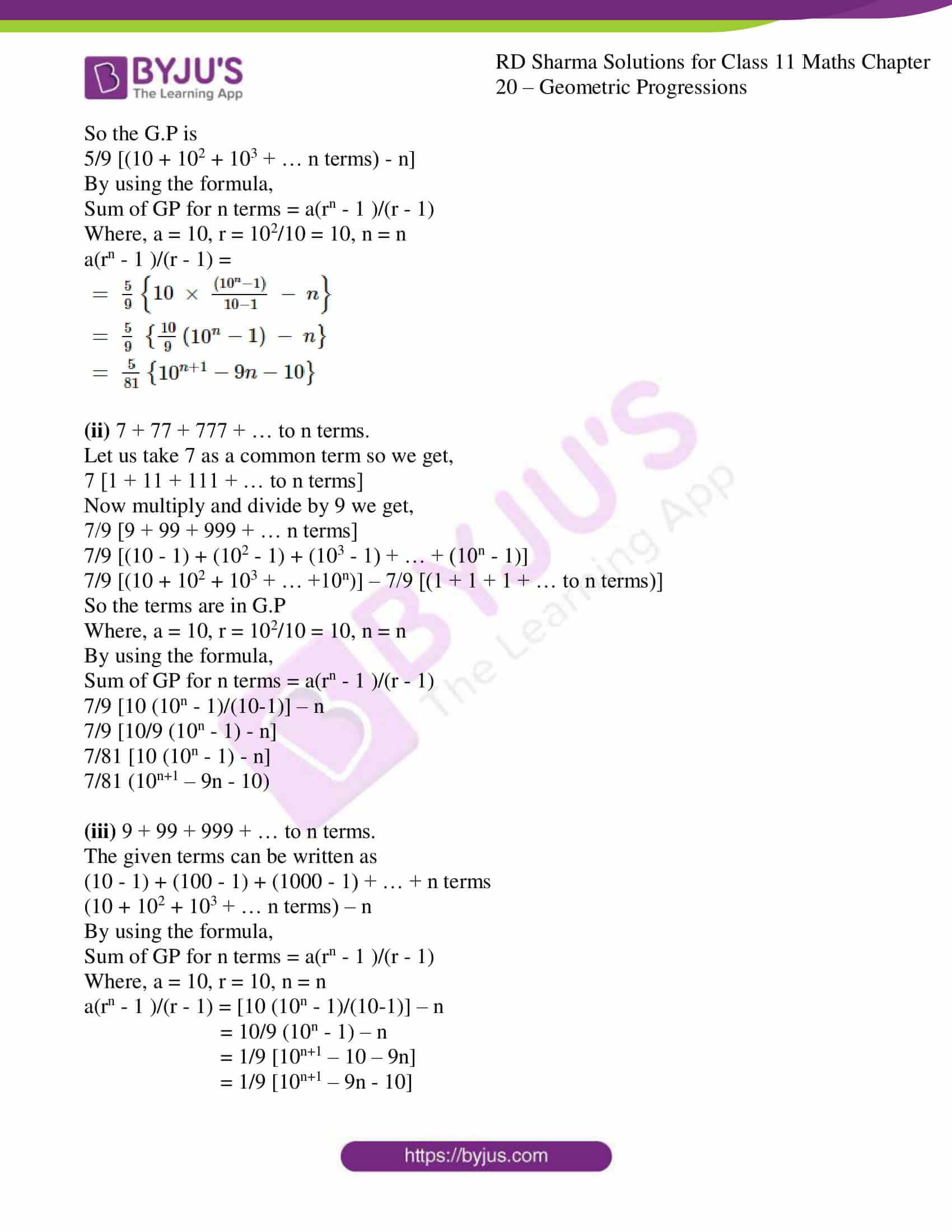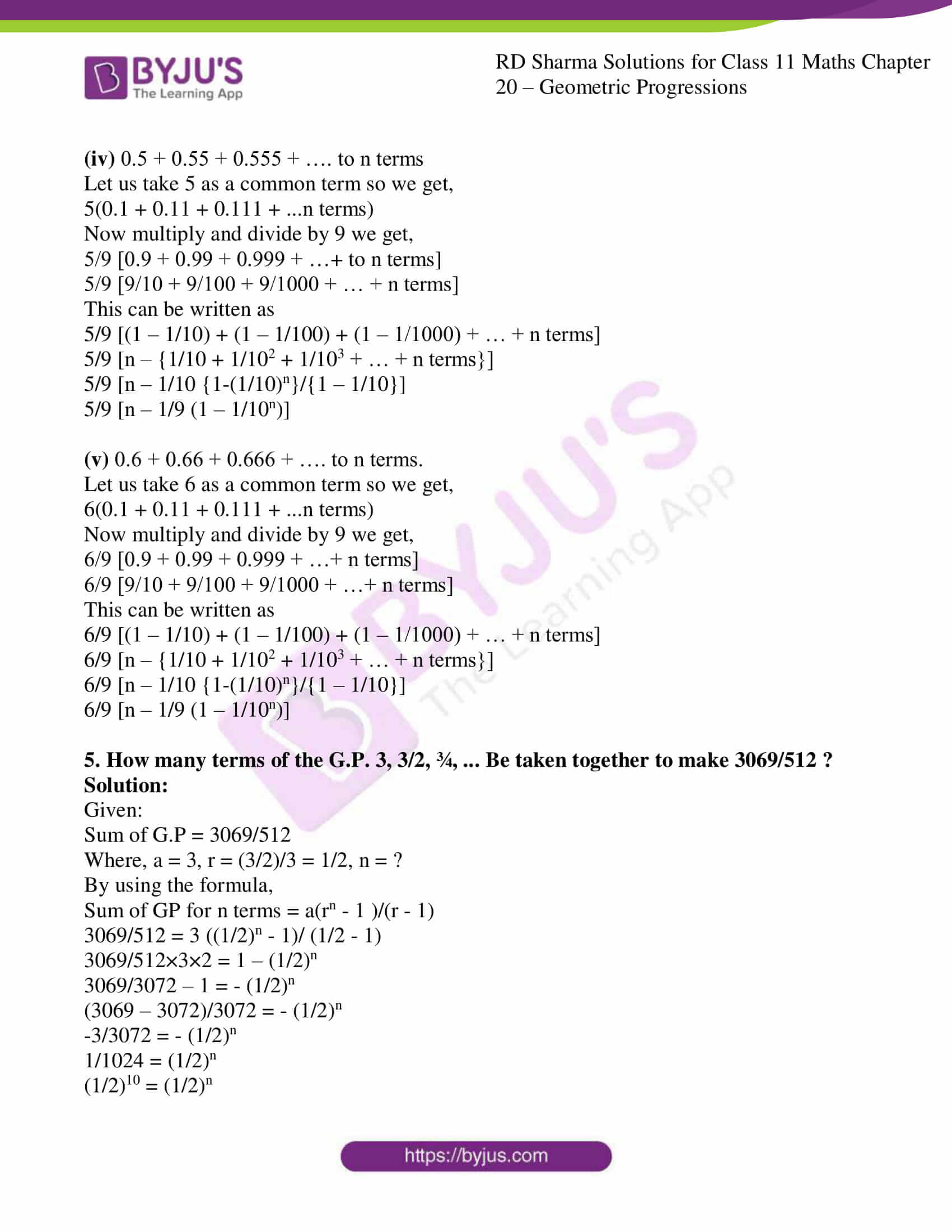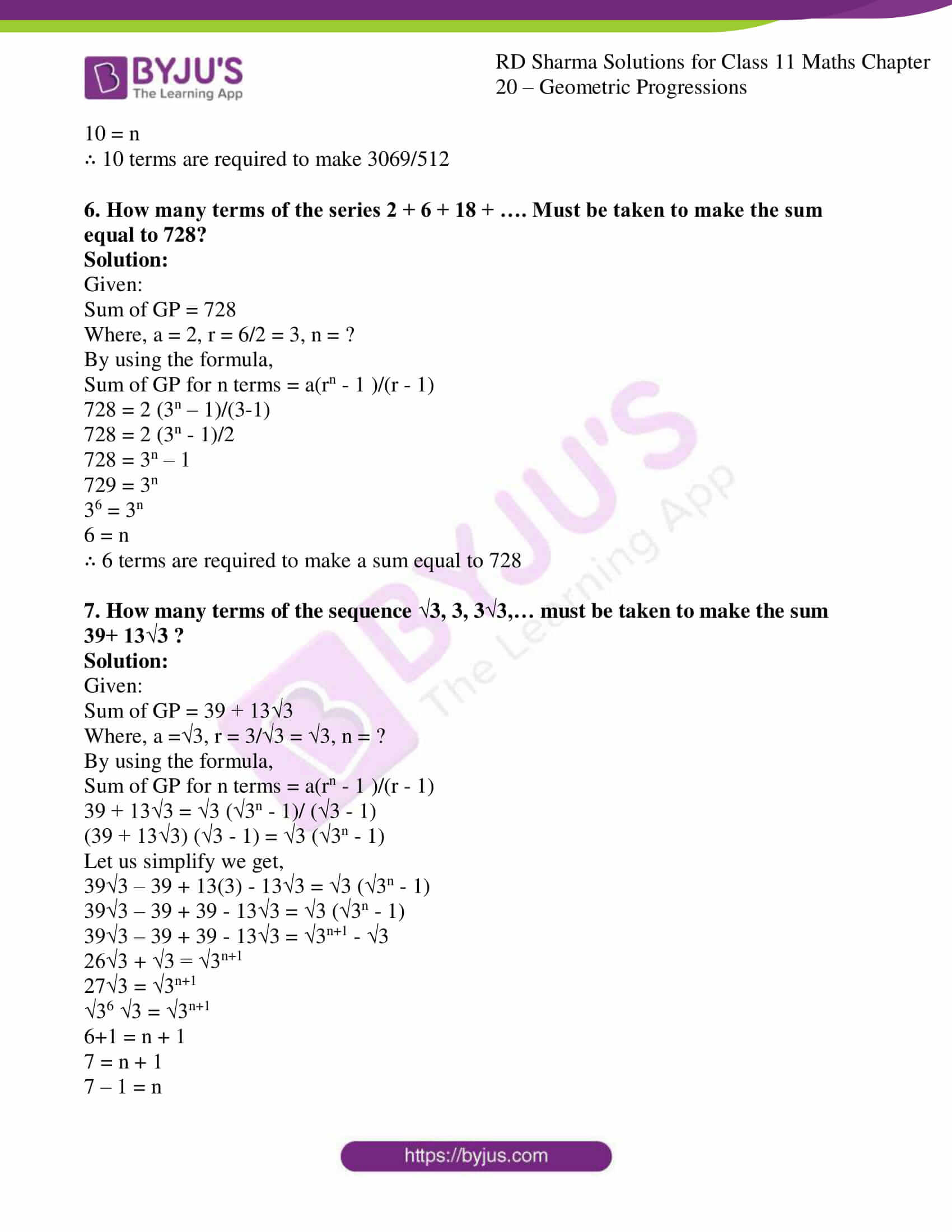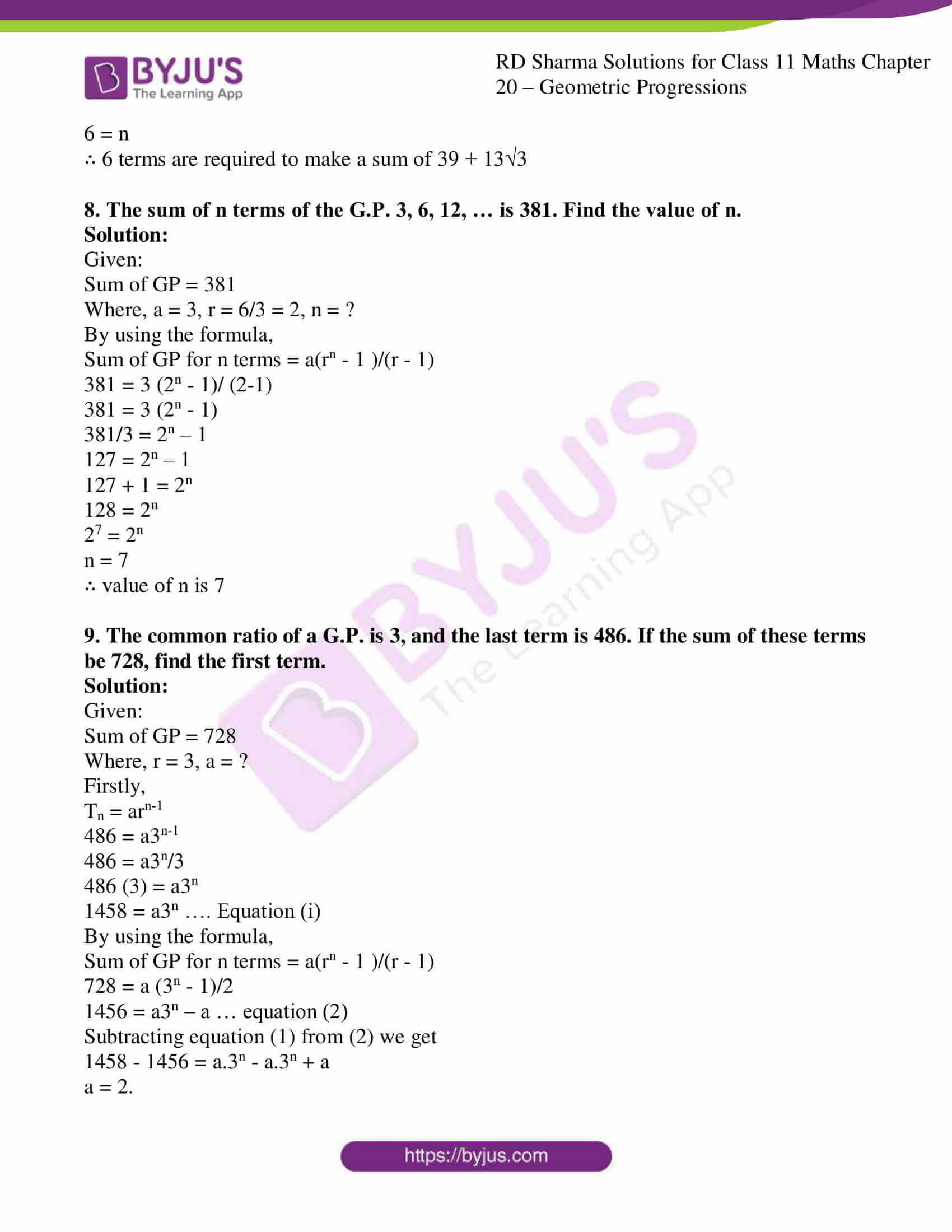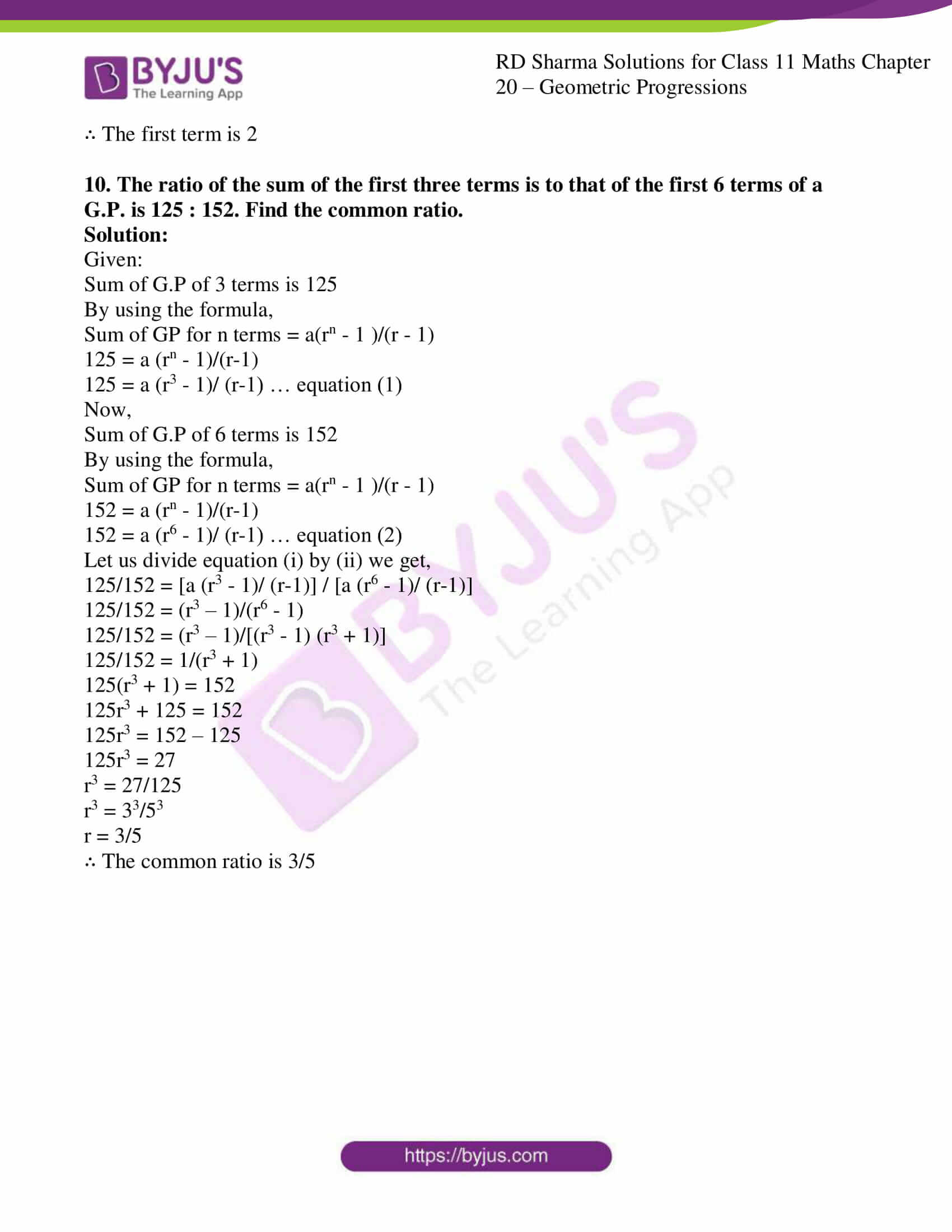### Also, access other exercises of RD Sharma Solutions for Class 11 Maths Chapter 20 – Geometric Progression

Exercise 20.1 Solutions

Exercise 20.2 Solutions

Exercise 20.4 Solutions

Exercise 20.5 Solutions

Exercise 20.6 Solutions

### Access answers to RD Sharma Solutions for Class 11 Maths Exercise 20.3 Chapter 20 – Geometric Progression

1. Find the sum of the following geometric progressions:

(i) 2, 6, 18, … to 7 terms

(ii) 1, 3, 9, 27, … to 8 terms

(iii) 1, -1/2, ¼, -1/8, …

(iv) (a2 – b2), (a – b), (a-b)/(a+b), … to n terms

(v) 4, 2, 1, ½ … to 10 terms

Solution:

(i) 2, 6, 18, … to 7 terms

We know that, sum of GP for n terms = a(rn – 1)/(r – 1)

Given:

a = 2, r = t2/t1 = 6/2 = 3, n = 7

Now let us substitute the values in

a(rn – 1)/(r – 1) = 2 (37 – 1)/(3-1)

= 2 (37 – 1)/2

= 37 – 1

= 2187 – 1

= 2186

(ii) 1, 3, 9, 27, … to 8 terms

We know that, sum of GP for n terms = a(rn – 1)/(r – 1)

Given:

a = 1, r = t2/t1 = 3/1 = 3, n = 8

Now let us substitute the values in

a(rn – 1)/(r – 1) = 1 (38 – 1)/(3-1)

= (38 – 1)/2

= (6561 – 1)/2

= 6560/2

= 3280

(iii) 1, -1/2, ¼, -1/8, …

We know that, sum of GP for infinity = a/(1 – r)

Given:

a = 1, r = t2/t1 = (-1/2)/1 = -1/2

Now let us substitute the values in

a/(1 – r) = 1/(1 – (-1/2))

= 1/(1 + 1/2)

= 1/((2+1)/2)

= 1/(3/2)

= 2/3

(iv) (a2 – b2), (a – b), (a-b)/(a+b), … to n terms

We know that, sum of GP for n terms = a(rn – 1)/(r – 1)

Given:

a = (a2 – b2), r = t2/t1 = (a-b)/(a2 – b2) = (a-b)/(a-b) (a+b) = 1/(a+b), n = n

Now let us substitute the values in

a(rn – 1)/(r – 1) =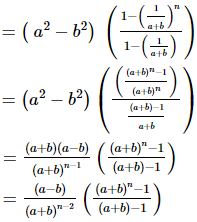(v) 4, 2, 1, ½ … to 10 terms

We know that, sum of GP for n terms = a(rn – 1)/(r – 1)

Given:

a = 4, r = t2/t1 = 2/4 = 1/2, n = 10

Now let us substitute the values in

a(rn – 1)/(r – 1) = 4 ((1/2)10 – 1)/((1/2)-1)

= 4 ((1/2)10 – 1)/((1-2)/2)

= 4 ((1/2)10 – 1)/(-1/2)

= 4 ((1/2)10 – 1) × -2/1

= -8 [1/1024 -1]

= -8 [1 – 1024]/1024

= -8 [-1023]/1024

= 1023/128

2. Find the sum of the following geometric series :
(i) 0.15 + 0.015 + 0.0015 + … to 8 terms;

(ii) √2 + 1/√2 + 1/2√2 + …. to 8 terms;

(iii) 2/9 – 1/3 + ½ – ¾ + … to 5 terms;

(iv) (x + y) + (x2 + xy + y2) + (x3 + x2 y + xy2 + y3) + …. to n terms ;

(v) 3/5 + 4/52 + 3/53 + 4/54 + … to 2n terms;

Solution:

(i) 0.15 + 0.015 + 0.0015 + … to 8 terms

Given:

a = 0.15

r = t2/t1 = 0.015/0.15 = 0.1 = 1/10

n = 8

By using the formula,

Sum of GP for n terms = a(1 – rn )/(1 – r)

a(1 – rn )/(1 – r) = 0.15 (1 – (1/10)8) / (1 – (1/10))

= 0.15 (1 – 1/108) / (1/10)

= 1/6 (1 – 1/108)

(ii) √2 + 1/√2 + 1/2√2 + …. to 8 terms;

Given:

a = √2

r = t2/t1 = (1/√2)/√2 = 1/2

n = 8

By using the formula,

Sum of GP for n terms = a(1 – rn )/(1 – r)

a(1 – rn )/(1 – r) = √2 (1 – (1/2)8) / (1 – (1/2))

= √2 (1 – 1/256) / (1/2)

= √2 ((256 – 1)/256) × 2

= √2 (255×2)/256

= (255√2)/128

(iii) 2/9 – 1/3 + ½ – ¾ + … to 5 terms;

Given:

a = 2/9

r = t2/t1 = (-1/3) / (2/9) = -3/2

n = 5

By using the formula,

Sum of GP for n terms = a(1 – rn )/(1 – r)

a(1 – rn )/(1 – r) = (2/9) (1 – (-3/2)5) / (1 – (-3/2))

= (2/9) (1 + (3/2)5) / (1 + 3/2)

= (2/9) (1 + (3/2)5) / (5/2)

= (2/9) (1 + 243/32) / (5/2)

= (2/9) ((32+243)/32) / (5/2)

= (2/9) (275/32) × 2/5

= 55/72

(iv) (x + y) + (x2 + xy + y2) + (x3 + x2 y + xy2 + y3) + …. to n terms;

Let Sn = (x + y) + (x2 + xy + y2) + (x3 + x2 y + xy2 + y3) + …. to n terms

Let us multiply and divide by (x – y) we get,

Sn = 1/(x – y) [(x + y) (x – y) + (x2 + xy + y2) (x – y) … upto n terms]

(x – y) Sn = (x2 – y2) + x3 + x2y + xy2 – x2y – xy2 – y3..upto n terms

(x – y) Sn = (x2 + x3 + x4+…n terms) – (y2 + y3 + y4 +…n terms)

By using the formula,

Sum of GP for n terms = a(1 – rn )/(1 – r)

We have two G.Ps in above sum, so,

(x – y) Sn = x2 [(xn – 1)/ (x – 1)] – y2 [(yn – 1)/ (y – 1)]

Sn = 1/(x-y) {x2 [(xn – 1)/ (x – 1)] – y2 [(yn – 1)/ (y – 1)]}

(v) 3/5 + 4/52 + 3/53 + 4/54 + … to 2n terms;

The series can be written as:

3 (1/5 + 1/53 + 1/55+ … to n terms) + 4 (1/52 + 1/54 + 1/56 + … to n terms)

Firstly let us consider 3 (1/5 + 1/53 + 1/55+ … to n terms)

So, a = 1/5

r = t2/t1 = 1/52 = 1/25

By using the formula,

Sum of GP for n terms = a(1 – rn )/(1 – r)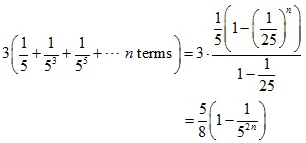Now, Let us consider 4 (1/52 + 1/54 + 1/56 + … to n terms)

So, a = 1/25

r = t2/t1 = 1/52 = 1/25

By using the formula,

Sum of GP for n terms = a(1 – rn )/(1 – r)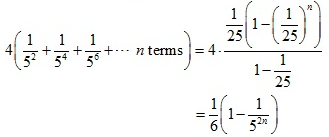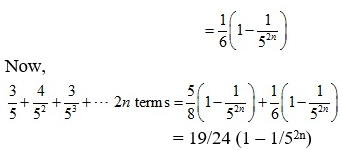3. Evaluate the following: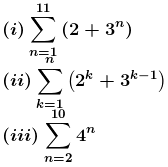Solution: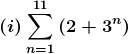= (2 + 31) + (2 + 32) + (2 + 33) + … + (2 + 311)

= 2×11 + 31 + 32 + 33 + … + 311

= 22 + 3(311 – 1)/(3 – 1) [by using the formula, a(1 – rn )/(1 – r)]

= 22 + 3(311 – 1)/2

= [44 + 3(177147 – 1)]/2

= [44 + 3(177146)]/2

= 265741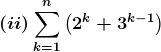= (2 + 30) + (22 + 3) + (23 + 32) + … + (2n + 3n-1)

= (2 + 22 + 23 + … + 2n) + (30 + 31 + 32 + …. + 3n-1)

Firstly let us consider,

(2 + 22 + 23 + … + 2n)

Where, a = 2, r = 22/2 = 4/2 = 2, n = n

By using the formula,

Sum of GP for n terms = a(rn – 1 )/(r – 1)

= 2 (2n – 1)/(2 – 1)

= 2 (2n – 1)

Now, let us consider

(30 + 31 + 32 + …. + 3n)

Where, a = 30 = 1, r = 3/1 = 3, n = n

By using the formula,

Sum of GP for n terms = a(rn – 1 )/(r – 1)

= 1 (3n – 1)/ (3 – 1)

= (3n – 1)/2

So,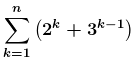= (2 + 22 + 23 + … + 2n) + (30 + 31 + 32 + …. + 3n)

= 2 (2n – 1) + (3n – 1)/2

= ½ [2n+2 + 3n – 4 – 1]

= ½ [2n+2 + 3n – 5]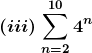= 42 + 43 + 44 + … + 410

Where, a = 42 = 16, r = 43/42 = 4, n = 9

By using the formula,

Sum of GP for n terms = a(rn – 1 )/(r – 1)

= 16 (49 – 1)/(4 – 1)

= 16 (49 – 1)/3

= 16/3 [49 – 1]

4. Find the sum of the following series :
(i) 5 + 55 + 555 + … to n terms.

(ii) 7 + 77 + 777 + … to n terms.

(iii) 9 + 99 + 999 + … to n terms.

(iv) 0.5 + 0.55 + 0.555 + …. to n terms

(v) 0.6 + 0.66 + 0.666 + …. to n terms.

Solution:

(i) 5 + 55 + 555 + … to n terms.

Let us take 5 as a common term so we get,

5 [1 + 11 + 111 + … n terms]

Now multiply and divide by 9 we get,

5/9 [9 + 99 + 999 + … n terms]

5/9 [(10 – 1) + (102 – 1) + (103 – 1) + … n terms]

5/9 [(10 + 102 + 103 + … n terms) – n]

So the G.P is

5/9 [(10 + 102 + 103 + … n terms) – n]

By using the formula,

Sum of GP for n terms = a(rn – 1 )/(r – 1)

Where, a = 10, r = 102/10 = 10, n = n

a(rn – 1 )/(r – 1) =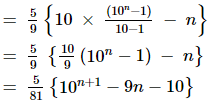(ii) 7 + 77 + 777 + … to n terms.

Let us take 7 as a common term so we get,

7 [1 + 11 + 111 + … to n terms]

Now multiply and divide by 9 we get,

7/9 [9 + 99 + 999 + … n terms]

7/9 [(10 – 1) + (102 – 1) + (103 – 1) + … + (10n – 1)]

7/9 [(10 + 102 + 103 + … +10n)] – 7/9 [(1 + 1 + 1 + … to n terms)]

So the terms are in G.P

Where, a = 10, r = 102/10 = 10, n = n

By using the formula,

Sum of GP for n terms = a(rn – 1 )/(r – 1)

7/9 [10 (10n – 1)/(10-1)] – n

7/9 [10/9 (10n – 1) – n]

7/81 [10 (10n – 1) – n]

7/81 (10n+1 – 9n – 10)

(iii) 9 + 99 + 999 + … to n terms.

The given terms can be written as

(10 – 1) + (100 – 1) + (1000 – 1) + … + n terms

(10 + 102 + 103 + … n terms) – n

By using the formula,

Sum of GP for n terms = a(rn – 1 )/(r – 1)

Where, a = 10, r = 10, n = n

a(rn – 1 )/(r – 1) = [10 (10n – 1)/(10-1)] – n

= 10/9 (10n – 1) – n

= 1/9 [10n+1 – 10 – 9n]

= 1/9 [10n+1 – 9n – 10]

(iv) 0.5 + 0.55 + 0.555 + …. to n terms

Let us take 5 as a common term so we get,

5(0.1 + 0.11 + 0.111 + …n terms)

Now multiply and divide by 9 we get,

5/9 [0.9 + 0.99 + 0.999 + …+ to n terms]

5/9 [9/10 + 9/100 + 9/1000 + … + n terms]

This can be written as

5/9 [(1 – 1/10) + (1 – 1/100) + (1 – 1/1000) + … + n terms]

5/9 [n – {1/10 + 1/102 + 1/103 + … + n terms}]

5/9 [n – 1/10 {1-(1/10)n}/{1 – 1/10}]

5/9 [n – 1/9 (1 – 1/10n)]

(v) 0.6 + 0.66 + 0.666 + …. to n terms.

Let us take 6 as a common term so we get,

6(0.1 + 0.11 + 0.111 + …n terms)

Now multiply and divide by 9 we get,

6/9 [0.9 + 0.99 + 0.999 + …+ n terms]

6/9 [9/10 + 9/100 + 9/1000 + …+ n terms]

This can be written as

6/9 [(1 – 1/10) + (1 – 1/100) + (1 – 1/1000) + … + n terms]

6/9 [n – {1/10 + 1/102 + 1/103 + … + n terms}]

6/9 [n – 1/10 {1-(1/10)n}/{1 – 1/10}]

6/9 [n – 1/9 (1 – 1/10n)]

5. How many terms of the G.P. 3, 3/2, ¾, … Be taken together to make 3069/512 ?

Solution:

Given:

Sum of G.P = 3069/512

Where, a = 3, r = (3/2)/3 = 1/2, n = ?

By using the formula,

Sum of GP for n terms = a(rn – 1 )/(r – 1)

3069/512 = 3 ((1/2)n – 1)/ (1/2 – 1)

3069/512×3×2 = 1 – (1/2)n

3069/3072 – 1 = – (1/2)n

(3069 – 3072)/3072 = – (1/2)n

-3/3072 = – (1/2)n

1/1024 = (1/2)n

(1/2)10 = (1/2)n

10 = n

∴ 10 terms are required to make 3069/512

6. How many terms of the series 2 + 6 + 18 + …. Must be taken to make the sum equal to 728?

Solution:

Given:

Sum of GP = 728

Where, a = 2, r = 6/2 = 3, n = ?

By using the formula,

Sum of GP for n terms = a(rn – 1 )/(r – 1)

728 = 2 (3n – 1)/(3-1)

728 = 2 (3n – 1)/2

728 = 3n – 1

729 = 3n

36 = 3n

6 = n

∴ 6 terms are required to make a sum equal to 728

7. How many terms of the sequence √3, 3, 3√3,… must be taken to make the sum 39+ 13√3 ?

Solution:

Given:

Sum of GP = 39 + 13√3

Where, a =√3, r = 3/√3 = √3, n = ?

By using the formula,

Sum of GP for n terms = a(rn – 1 )/(r – 1)

39 + 13√3 = √3 (√3n – 1)/ (√3 – 1)

(39 + 13√3) (√3 – 1) = √3 (√3n – 1)

Let us simplify we get,

39√3 – 39 + 13(3) – 13√3 = √3 (√3n – 1)

39√3 – 39 + 39 – 13√3 = √3 (√3n – 1)

39√3 – 39 + 39 – 13√3 = √3n+1 – √3

26√3 + √3 = √3n+1

27√3 = √3n+1

√36 √3 = √3n+1

6+1 = n + 1

7 = n + 1

7 – 1 = n

6 = n

∴ 6 terms are required to make a sum of 39 + 13√3

8. The sum of n terms of the G.P. 3, 6, 12, … is 381. Find the value of n.

Solution:

Given:

Sum of GP = 381

Where, a = 3, r = 6/3 = 2, n = ?

By using the formula,

Sum of GP for n terms = a(rn – 1 )/(r – 1)

381 = 3 (2n – 1)/ (2-1)

381 = 3 (2n – 1)

381/3 = 2n – 1

127 = 2n – 1

127 + 1 = 2n

128 = 2n

27 = 2n

n = 7

∴ value of n is 7

9. The common ratio of a G.P. is 3, and the last term is 486. If the sum of these terms be 728, find the first term.

Solution:

Given:

Sum of GP = 728

Where, r = 3, a = ?

Firstly,

Tn = arn-1

486 = a3n-1

486 = a3n/3

486 (3) = a3n

1458 = a3n …. Equation (i)

By using the formula,

Sum of GP for n terms = a(rn – 1 )/(r – 1)

728 = a (3n – 1)/2

1456 = a3n – a … equation (2)

Subtracting equation (1) from (2) we get

1458 – 1456 = a.3n – a.3n + a

a = 2.

∴ The first term is 2

10. The ratio of the sum of the first three terms is to that of the first 6 terms of a G.P. is 125 : 152. Find the common ratio.

Solution:

Given:

Sum of G.P of 3 terms is 125

By using the formula,

Sum of GP for n terms = a(rn – 1 )/(r – 1)

125 = a (rn – 1)/(r-1)

125 = a (r3 – 1)/ (r-1) … equation (1)

Now,

Sum of G.P of 6 terms is 152

By using the formula,

Sum of GP for n terms = a(rn – 1 )/(r – 1)

152 = a (rn – 1)/(r-1)

152 = a (r6 – 1)/ (r-1) … equation (2)

Let us divide equation (i) by (ii) we get,

125/152 = [a (r3 – 1)/ (r-1)] / [a (r6 – 1)/ (r-1)]

125/152 = (r3 – 1)/(r6 – 1)

125/152 = (r3 – 1)/[(r3 – 1) (r3 + 1)]

125/152 = 1/(r3 + 1)

125(r3 + 1) = 152

125r3 + 125 = 152

125r3 = 152 – 125

125r3 = 27

r3 = 27/125

r3 = 33/53

r = 3/5

∴ The common ratio is 3/5